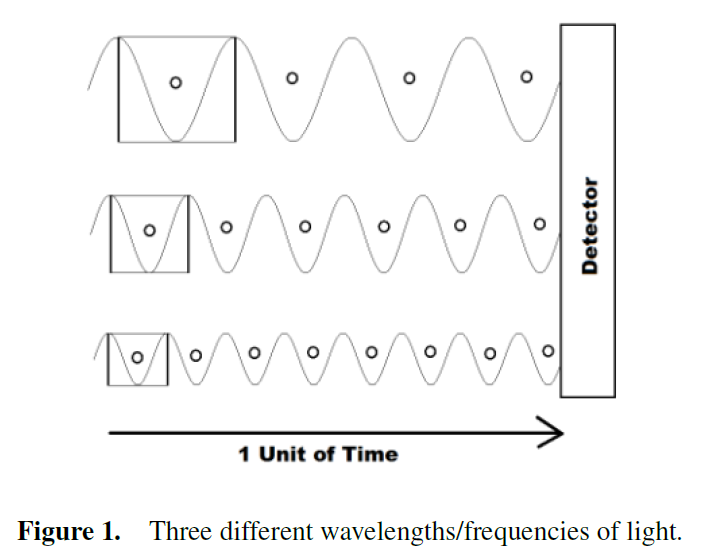# Cycles, Planck's Constant, and the True Nature of Light

in MES Science2 years ago (edited)

Lori Gardi aka Fractal Woman has undergone a close examination of the conventional physics of light and has shown that the fundamental units used greatly affects the resulting interpretations.

The mainstream convention when describing light is by using terms such as "frequency" in units of 1/s or 1/second and thus ignoring the "cycles" part. This leads to interpreting the famous equation E = h f (where h is Planck's constant and f is the frequency) as representing the "quantum of energy in a single photon-light particle of frequency f".

But instead of ignoring the "cycles" term in f, and adopting Fractal Woman's Modified Unit Analysis technique, then including the cycle term properly interprets the actual quantum as being Planck's constant itself and thus there is no "photon particle"! This means that all light waves have the same amount of "energy per cycle" and thus "higher frequency gamma waves" are not distinct "photons" but rather just have more of the same "Planck's quanta of energy". This is some truly ground-breaking science here!

Here is her 2018 video discussing this:

Here is a recent update as well:

Here is a link to her associated paper for reference:Sort: